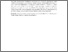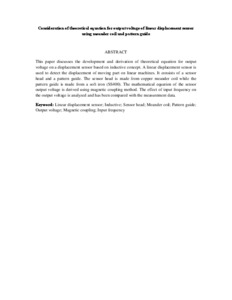# Consideration of theoretical equation for output voltage of linear displacement sensor using meander coil and pattern guide

## Citation

Misron, Norhisam and Abdullah, Norrimah and Wagiran, Rahman and Mohd Sidek, Roslina and Mariun, Norman and Wakiwaka, Hiroyuki (2008) Consideration of theoretical equation for output voltage of linear displacement sensor using meander coil and pattern guide. Sensors and Actuators A: Physical, 147 (2). pp. 470-473. ISSN 0924-4247; ESSN: 1873-3069

## Abstract

This paper discusses the development and derivation of theoretical equation for output voltage on a displacement sensor based on inductive concept. A linear displacement sensor is used to detect the displacement of moving part on linear machines. It consists of a sensor head and a pattern guide. The sensor head is made from copper meander coil while the pattern guide is made from a soft iron (SS400). The mathematical equation of the sensor output voltage is derived using magnetic coupling method. The effect of input frequency on the output voltage is analyzed and has been compared with the measurement data.Preview
Text (Abstract)
Consideration of theoretical equation for output voltage of linear displacement sensor using meander coil and pattern guide.pdfView Item